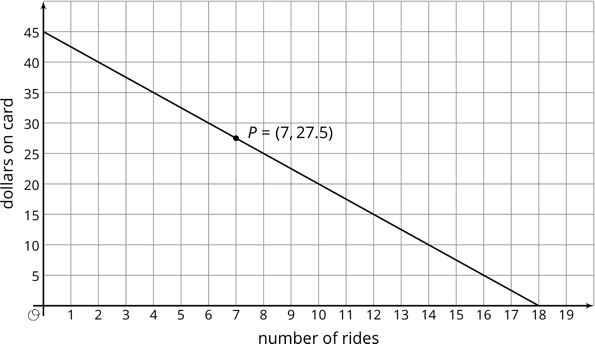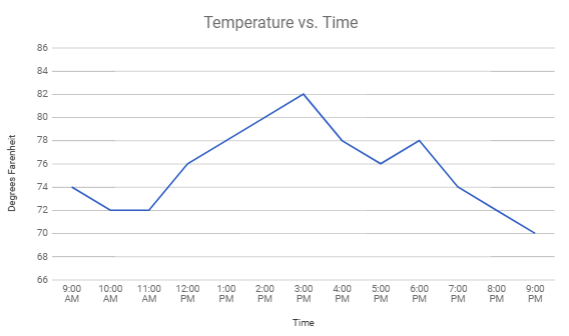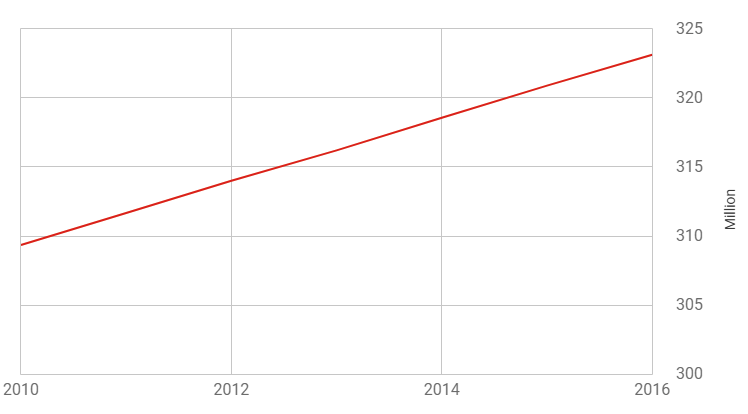# Functions

## Objective

Identify properties of functions represented in graphs.

## Common Core Standards

### Core Standards

?

• 8.F.A.1 — Understand that a function is a rule that assigns to each input exactly one output. The graph of a function is the set of ordered pairs consisting of an input and the corresponding output. Function notation is not required in Grade 8.

• 8.F.B.4 — Construct a function to model a linear relationship between two quantities. Determine the rate of change and initial value of the function from a description of a relationship or from two (x, y) values, including reading these from a table or from a graph. Interpret the rate of change and initial value of a linear function in terms of the situation it models, and in terms of its graph or a table of values.

?

• 7.RP.A.2.B

• 7.RP.A.2.D

## Criteria for Success

?

1. Find a rate of change of a function represented in a graph.
2. Find the initial value of a function represented in a graph.
3. Interpret inputs and outputs of graphs in context (MP.2).
4. Determine if a set of ordered pairs from a graph represent a function.

## Tips for Teachers

?

In this lesson, students further their analysis of a function graph to find rate of change and initial value. In seventh grade, students found the constant of proportionality in proportional graphs. This lesson prepares students to compare functions across multiple representations in upcoming lessons in this unit, and to determine slope of linear functions in Unit 5.

#### Fishtank Plus

• Problem Set
• Student Handout Editor
• Vocabulary Package

## Anchor Problems

?

### Problem 1

The graph below shows the amount of money on a subway card as a function of the number of subway rides taken. Use the graph to answer the questions that follow.1. After 2 rides, how much money is on the card?
2. After 4 rides, how much money is on the card?
3. At what rate is the money on the card changing for each ride taken?
4. How much money did the card initially have on it?
5. Interpret point P in context of the situation.

#### References

Open Up Resources Teacher Version: Grade 8 Unit 5 Lesson 44.4 Cool Down

Modified by The Match Foundation, Inc.

### Problem 2

In a laboratory, a scientist is tracking the temperature of a substance over time. Each hour, she takes the temperature and records it in the graph shown below.1. What is the rate of change of the substance’s temperature, in °F per hour, between 12 PM and 3 PM?
2. What is the rate of change of the substance’s temperature, in °F per hour, between 7 PM and 9 PM?
3. What is the starting temperature of the substance?
4. Does it appear that the temperature of the substance is a function of the time of day? Why or why not?

### Problem 3

The ordered pairs below are from a graph, which is not shown. Based on the ordered pairs, can you determine if the graph is a function?

${(3,4)}$   ${(2,5)}$   ${(1,6)}$   ${(0,7)}$   ${(1,8)}$

## Problem Set

?The following resources include problems and activities aligned to the objective of the lesson that can be used to create your own problem set.

• Include images of graphs, and ask students to
• Find outputs based on inputs (and vice versa).
• Find a rate of change (in general for linear relationships, or between two given inputs for a nonlinear relationship).
• Find an initial value (if relevant).
• Contexts for graphs could include distance traveled over time, speed over time, temperature over time, costs over time, etc.
• Include examples of lists of coordinate points and ask students to determine if the relationship represents a function.

?

The graph below shows the population of the United States over time using data from the U.S. Census Buraeu.1. Approximately what was the population of the United States in 2010, 2012, and 2014?
2. Approximately when did the population of the United States pass 310 million people?
3. Between 2012 and 2014, what was the rate of change of the population in the United States?
4. Describe the relationship as a function.

#### References

U.S. Census Bureau, Population Division Website: Annual Estimates of the Residential Population: April 1, 2010 to July 1, 2016

Annual Estimates of the Residential Population: April 1, 2010 to July 1, 2016 is made available by the U.S. Census Bureau. Accessed Oct. 31, 2017, 3:51 p.m..

?